How to use CUDA web apps

You can access the project site through the link:They are:

1.     Matrix Operations

2.     Mandelbrot

3.     Sorting

4.     Generating Random Matrices

You could able to do the above operations within a very short time with the parallel programing used in this project.

Following paragraphs will explain how to use the calculation methods available under the above topics.

1.     Matrix Operations

In “Matrix Operations”, there are seven options available.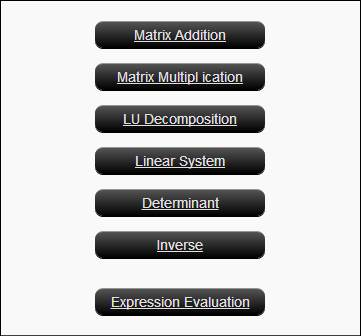You have to upload two matrix files (in .txt format) and give the number of rows and number of columns. Then click, Send Request to get the answer.ii.            Matrix Multiplication

Here also you have to upload two matrices (in .txt format). Here you have to give the number of rows and number of columns to both matrices. Then click, Send Request to get the answer.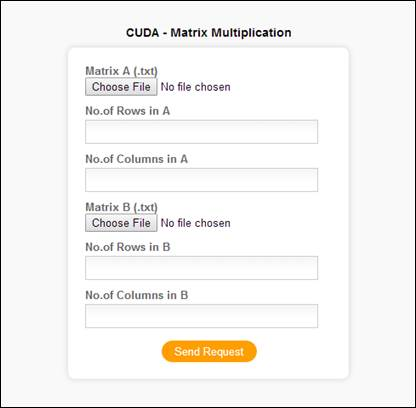iii.LU Decomposition

Upload a square matrix (in .txt format) and give number of rows/columns. Then click, Send Request to get the answer.iv.            Linear System

Here you have to upload two files (in .txt format), one is square matrix and other one is a vector(single column matrix) with the same number of rows in the square matrix. Also, give the number of rows in the matrices. Then click, Send Request to get the answer.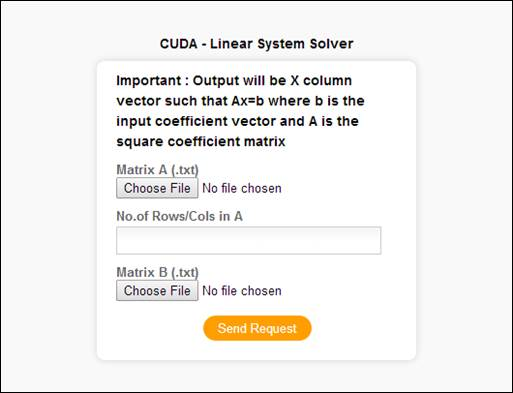v.            Determinant

Upload a square matrix (in .txt format) and give the number of rows. Then click, Send Request to get the answer.vi.            Inverse

Upload a square matrix (in .txt format) and give the number of rows. Then click, Send Request to get the answer.vii.            Expression Evaluation

This is the big and last part under matrix operations, here you can upload up to five matrices and there you have to input number of columns  and rows in each uploaded matrix.

In the expression area, you have to provide an equation to operate on the matrices you gave.

There are some instructions available to you at the page itself also.

-         Expressions can have +,-,* operations including brackets.

-         Inverse can be stated like inv (A) But nested inverses like inv (A*inv (B)) or inv (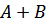) are not supported.

-         Spaces are allowed. But not mandatory.

-         Up to 5 matrices are supported. Keep unnecessary ones blank.2.     Mandelbrot

In “Mandelbrot”, you have to give six inputs. They are:

1.  Width

2.  Height

3.  Real Minimum

4.  Real Maximum

5.  Imaginary Minimum

6.  Imaginary Maximum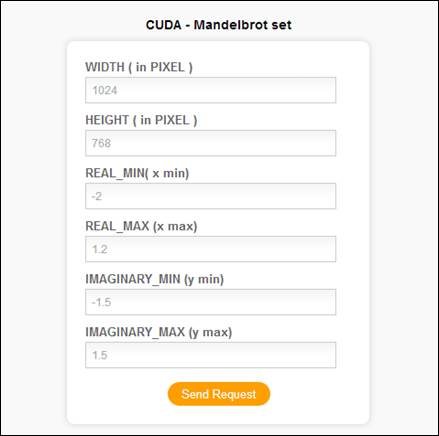Some example outputs of Mandelbrot set:3.     Sorting

In “Sorting”, you have to upload one file containing a list and give the number of elements in the list. Then click, Send Request to get the answer.4.     Random Matrix

In “Random Matrix”, you have to give the number of columns and number of rows. Then click, Send Request to get the answer.See the FAQ section for problems you encounter.